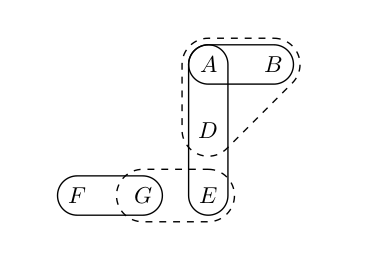# Drawing Hypergraph using TikZ

I want to draw a Hypergraph using TikZ that looks like thisWhat I have so far:

\usepackage{tikz}
\usetikzlibrary{calc, positioning, fit, shapes.misc}

\begin{document}
\begin{tikzpicture}
[
he/.style={draw, rounded corners},        % he = hyper edge
ce/.style={draw, dashed, rounded corners} % ce = condition edge
]

\node (f) at (0,0) {$F$};
\node (g) at (1,0) {$G$};
\node (e) at (2,0) {$E$};
\node (d) at (2,1) {$D$};
\node (a) at (2,2) {$A$};
\node (b) at (3,2) {$B$};

\node [he, fit=(f) (g)] {};
\node [ce, fit=(g) (e)] {};
\node [he, fit=(a) (d) (e)] {};
\node [ce, fit=(a) (b) (d)] {};

\end{tikzpicture}
\end{document}I didn't manage to ..

• Make the hyperedges a little bit smaller like in the original picture, so that you can distinguish between hyperedges and condition edges
• Make the ABD condition edge a triangle with rounded corners

Do you have any ideas how to do this? Furthermore, this is my first time working with TikZ, so if you have any other recommendations on how to improve the way of creating this graph and how to define styles, I would be happy to learn more.

• you can give inner sep=0pt in style definition of he to make hyper edges smaller Oct 28 '18 at 12:11
• Thanks @nidhin. So far I tried to adjust the box sizes with scale, but inner sep works better.
– ncw
Oct 28 '18 at 12:16

You can change the inner sep of hyper edge or conditional edge so that they both will have different sizes.

I don't have a good solution for the second part using fit library. I just drew that conditional edge.\documentclass{article}
\usepackage{tikz}
\usetikzlibrary{calc, positioning, fit, shapes.misc}

\begin{document}
\begin{tikzpicture}
[
he/.style={draw, rounded corners,inner sep=0pt},        % he = hyper edge
ce/.style={draw,dashed, rounded corners=10pt}, % ce = condition edge
]

\node (f) at (0,0) {$F$};
\node (g) at (1,0) {$G$};
\node (e) at (2,0) {$E$};
\node (d) at (2,1) {$D$};
\node (a) at (2,2) {$A$};
\node (b) at (3,2) {$B$};

\node [he, fit=(f) (g)] {};
\node [ce, fit=(g) (e)] {};
\node [he, fit=(a) (d) (e)] {};
\node [fit=(a) (b) (d)] (fd){};
\draw [dashed,rounded corners=10pt] ($(fd.south west)+(0,-0.5)$) -- (fd.north west) -- ($(fd.north east)+(0.5,0)$)--cycle;
\end{tikzpicture}
\end{document}

• I was just about to figure out how to draw a triangle around the nodes like you did, but I hard-coded the coordinates of the corners which is very tedious if I would ever want to add another triangle to the graph again. Your solution looks fine, thanks!
– ncw
Oct 28 '18 at 12:41

You can use my (semi) library (https://gitlab.com/gruenwald/tikz-hypergraphs) that I made for exactly this purpose:

\documentclass{article}
\usepackage{tikz}
\usepackage{ifthen}             % For tikz-hypergraph
\usetikzlibrary{calc}           % For tikz-hypergraph
\input{tikz-hypergraph}

\begin{document}
\begin{tikzpicture}
[
he/.style={draw, semithick},        % he = hyper edge
ce/.style={draw, dashed, semithick}, % ce = condition edge
]

\node (f) at (0,0) {$F$};
\node (g) at (1,0) {$G$};
\node (e) at (2,0) {$E$};
\node (d) at (2,1) {$D$};
\node (a) at (2,2) {$A$};
\node (b) at (3,2) {$B$};

\draw[he] \hedgeii{a}{b}{3mm};
\draw[he] \hedgeii{a}{e}{3mm};
\draw[ce] \hedgeiii{a}{b}{d}{4mm};
\draw[ce] \hedgeii{g}{e}{4mm};
\draw[he] \hedgeii{f}{g}{3mm};

\end{tikzpicture}
\end{document}